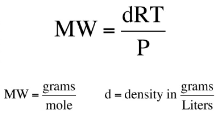# Problem: The air in a hot-air balloon at 744 torr is heated from 17°C to 60.0°C. Assuming that the amount (mol) of air and the pressure remain constant, what is the density of the air at each temperature? (The average molar mass of air is 28.8 g/mol.)

###### FREE Expert Solution

We can use the molar weight derivative of the ideal gas law for this problem :Set up the equation that had d isolated:

90% (299 ratings)###### Problem Details

The air in a hot-air balloon at 744 torr is heated from 17°C to 60.0°C. Assuming that the amount (mol) of air and the pressure remain constant, what is the density of the air at each temperature? (The average molar mass of air is 28.8 g/mol.)